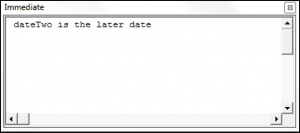### VBA Date Functions

In this tutorial, we are going to go through the different built-in VBA Date Functions.

### VBA Date Function

You can use the Date Function to return the current date.

The syntax of the Date Function is Date(). It has no arguments.

The following code shows you how to use the Date Function:

The result is: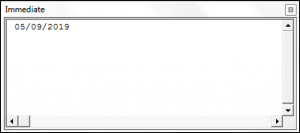### VBA Now Function

You can use the Now Function to return the current date and time.

The syntax of the Now Function is Now(). It has no arguments.

The following code shows you how to use the Now Function:

The result is: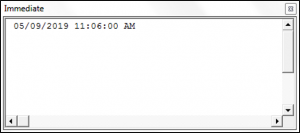### VBA Time Function

You can use the Time Function to return the current time.

The syntax of the Time Function is Time(). It has no arguments.

The following code shows you how to use the Time Function:

The result is: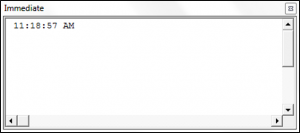You can use the DateAdd Function to add a date/time interval to a date or time, and the function will return the resulting date/time.

The syntax of the DateAdd Function is:

• Interval – A string that specifies the type of interval to use. The interval can be one of the following values:

“d” – day
“ww” – week
“w” – weekday
“m” – month
“q” – quarter
“yyyy” – year
“y” – day of the year
“h” – hour
“n” – minute
“s” – second

• Number – The number of intervals that you want to add to the original date/time.
• Date – The original date/time.

Note: When using dates in your code you have to surround them with # or quotation marks.

The following code shows  how to use the DateAdd Function:

The result is: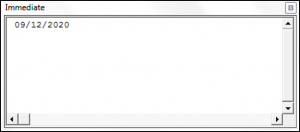### VBA DateDiff Function

You can use the DateDiff Function in order to get the difference between two dates, based on a specified time interval.

The syntax of the DateDiff Function is:

DateDiff(Interval, Date1, Date2, [Firstdayofweek], [Firstweekofyear]) where:

• Interval – A string that specifies the type of interval to use. The interval can be one of the following values:

“d” – day
“ww” – week
“w” – weekday
“m” – month
“q” – quarter
“yyyy” – year
“y” – day of the year
“h” – hour
“n” – minute
“s” – second

• Date1 – A date value representing the earlier date.
• Date2 – A date value representing the later date.
• Firstdayofweek (Optional) – A constant that specifies the weekday that the function should use as the first day of the week. If blank Sunday is used as the first day of the week. Firstdayofweek can be one of the following values:

-vbSunday – uses Sunday as the first day of the week.
-vbMonday – uses Monday as the first day of the week.
-vbTuesday – uses Tuesday as the first day of the week.
-vbWednesday – uses Wednesday as the first day of the week.
-vbThursday – uses Thursday as the first day of the week.
-vbFriday – uses Friday as the first day of the week.
-vbSaturday – uses Saturday as the first day of the week.
-vbUseSystemDayOfTheWeek – uses the first day of the week that is specified by your system’s settings.

• Firstweekofyear (Optional) – A constant that specifies the first week of the year. If blank then the Jan 1st week is used as the first week of the year. Firstweekofyear can be one of the following values:

-vbFirstJan1 – uses the week containing Jan 1st.
-vbFirstFourDays – uses the first week that contains at least four days in the new year.
-vbFirstFullWeek – uses the first full week of the year.
-vbSystem – uses the first week of the year as specified by your system settings.

The following code shows you how to use the DateDiff Function:

The result is: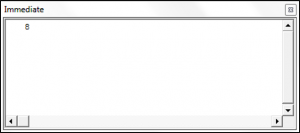### VBA DatePart Function

You can use the DatePart Function in order to return a part (day, week, quarter, month etc.) of a given date.

The syntax of the DatePart Function is:

DatePart(Interval, Date,[Firstdayofweek], [Firstweekofyear]) where:

• Interval – A string that specifies the part of the date to return. The interval can be one of the following values:

“d” – day
“ww” – week
“w” – weekday
“m” – month
“q” – quarter
“yyyy” – year
“y” – day of the year
“h” – hour
“n” – minute
“s” – second

• Date – The date that you want the function to return a part of.
• Firstdayofweek (Optional) – A constant that specifies the weekday that the function should use as the first day of the week. If blank Sunday is used as the first day of the week. Firstdayofweek can be one of the following values:

-vbSunday – uses Sunday as the first day of the week.
-vbMonday – uses Monday as the first day of the week.
-vbTuesday – uses Tuesday as the first day of the week.
-vbWednesday – uses Wednesday as the first day of the week.
-vbThursday – uses Thursday as the first day of the week.
-vbFriday – uses Friday as the first day of the week.
-vbSaturday – uses Saturday as the first day of the week.
-vbUseSystemDayOfTheWeek – uses the first day of the week that is specified by your system’s settings.

• Firstweekofyear (Optional) – A constant that specifies the first week of the year. If blank then the Jan 1st week is used as the first week of the year. Firstweekofyear can be one of the following values:

-vbFirstJan1 – uses the week containing Jan 1st.
-vbFirstFourDays – uses the first week that contains at least four days in the new year.
-vbFirstFullWeek – uses the first full week of the year.
-vbSystem – uses the first week of the year as specified by your system settings.

The following code shows you how to use the DatePart Function:

The result is: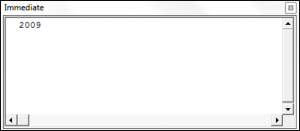### VBA DateSerial Function

The VBA DateSerial Function takes an input year, month and day and returns a date.

The syntax of the DateSerial Function is:

DateSerial(Year,  Month, Day) where:

• Year – An integer value between 100 and 9999 that represents the year.
• Month – An integer value that represents the month.
• Day – An integer value that represents the day.

The following code shows you how to use the DateSerial Function:

The result is: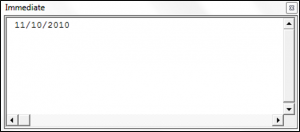### VBA DateValue Function

The DateValue Function returns a Date when given a string representation of a date.

The syntax of the DateValue Function is:

DateValue(Date) where:

• Date – A String representing the date.

The following code shows you how to use the DateValue Function:

The result is: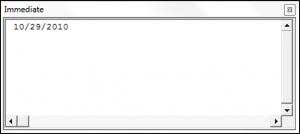### VBA Day Function

You can use the Day Function to return the day of an input date.

The syntax of the Day Function is:

Day(Date_value) where:

• Date_value – The date which you want to extract the day from.

The following code shows you how to use the Day Function:

The result is: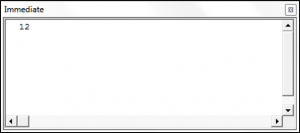### VBA Hour Function

You can use the Hour Function to return the hour of an input time.

The syntax of the Hour Function is:

Hour(Time) where:

• Time – The time that you want to extract the hour from.

The following code shows you how to use the Hour Function:

The result is: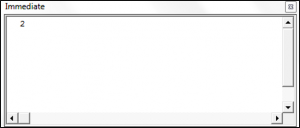### VBA Minute Function

You can use the Minute Function to return the minute value of an input time.

The syntax of the Minute Function is:

Minute(Time) where:

• Time – The time that you want to extract the minute value from.

The following code shows you how to use the Minute Function:

The result is: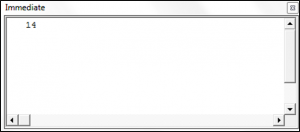### VBA Second Function

You can use the Second Function to return the second value of an input time.

The syntax of the Second Function is:

Second(Time) where:

• Time – The time that you want to extract the second value from.

The following code shows you how to use the Second Function:

The result is: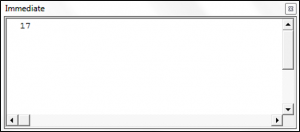### VBA Month Function

You can use the Month Function to return the month of an input date.

The syntax of the Month Function is:

Month(Date_value) where:

• Date_value – The date which you want to extract the month from.

The following code shows you how to use the Month Function:

The result is: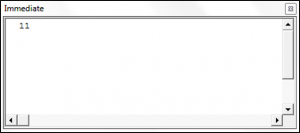### VBA MonthName Function

You can use the MonthName Function to return the name of a month from an input supplied month number.

The syntax of the MonthName Function is:

MonthName(Number_of_month, [Abbreviate]) where:

• Number_of_month – An integer value between 1 and 12.
• Abbreviate (Optional) – Specifies whether the month name should be abbreviated. If blank the default value of False is used.

The result is: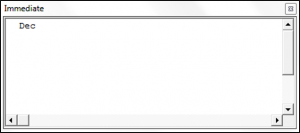### VBA TimeSerial Function

The TimeSerial Function takes an input hour, minute and second and returns a time.

The syntax of the TimeSerial Function is:

TimeSerial(Hour,  Minute, Second) where:

• Hour – An integer value between 0 and 23 that represents the hour value.
• Minute – An integer value between 0 and 59 that represents the minute value.
• Second – An integer value between 0 and 59 that represents the second value.

The following code shows you how to use the TimeSerial Function:

The result is: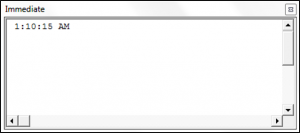### VBA TimeValue Function

The TimeValue Function returns a Time from a string representation of a date or time.

The syntax of the TimeValue Function is:

TimeValue(Time) where:

• Time – A String representing the time.

The following code shows you how to use the TimeValue Function:

The result is: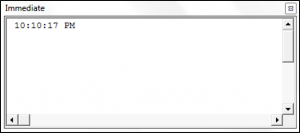### VBA Weekday Function

You can use the Weekday Function to return an integer from 1 – 7 representing a day of the week from an input date.

The syntax of the Weekday Function is:

Weekday(Date, [Firstdayofweek]) where:

• Date – The date that you want to extract the weekday value from.
• Firstdayofweek (Optional) – A constant that specifies the weekday that the function should use as the first day of the week. If blank Sunday is used as the first day of the week. Firstdayofweek can be one of the following values:

-vbSunday – uses Sunday as the first day of the week.
-vbMonday – uses Monday as the first day of the week.
-vbTuesday – uses Tuesday as the first day of the week.
-vbWednesday – uses Wednesday as the first day of the week.
-vbThursday – uses Thursday as the first day of the week.
-vbFriday – uses Friday as the first day of the week.
-vbSaturday – uses Saturday as the first day of the week.
-vbUseSystemDayOfTheWeek – uses the first day of the week that is specified by your system’s settings.

The following code shows you how to use the Weekday Function:

The result is: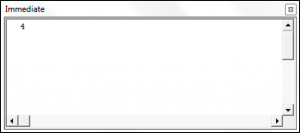### VBA WeekdayName Function

You can use the WeekdayName Function to return the name of a weekday from an input supplied weekday number.

The syntax of the WeekdayName Function is:

WeekdayName(Weekday, [Abbreviate], [Firstdayoftheweek]) where:

• Weekday – An integer value between 1 and 7.
• Abbreviate (Optional) -Specifies whether the weekday name should be abbreviated. If blank the default value of False is used.
• Firstdayofweek (Optional) – A constant that specifies the weekday that the function should use as the first day of the week. If blank Sunday is used as the first day of the week. Firstdayofweek can be one of the following values:

-vbSunday – uses Sunday as the first day of the week.
-vbMonday – uses Monday as the first day of the week.
-vbTuesday – uses Tuesday as the first day of the week.
-vbWednesday – uses Wednesday as the first day of the week.
-vbThursday – uses Thursday as the first day of the week.
-vbFriday – uses Friday as the first day of the week.
-vbSaturday – uses Saturday as the first day of the week.
-vbUseSystemDayOfTheWeek – uses the first day of the week that is specified by your system’s settings.

The result is: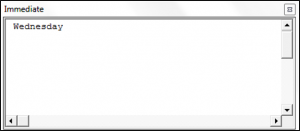### VBA Year Function

You can use the Year Function to return the year of an input date.

The syntax of the Year Function is:

Year(Date_value) where:

• Date_value – The date which you want to extract the year from.

The following code shows you how to use the Year Function:

The result is: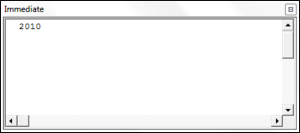### Comparing Dates in VBA

You can compare dates using the >, <, and = operators in VBA. The following code shows you how to compare two dates in VBA.